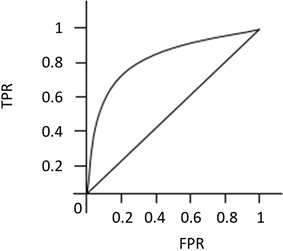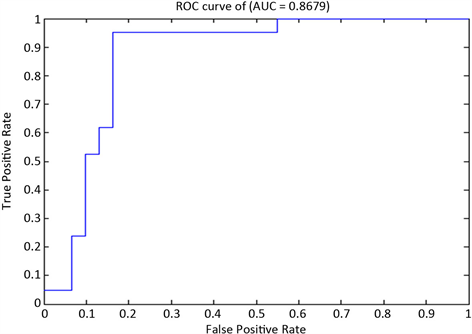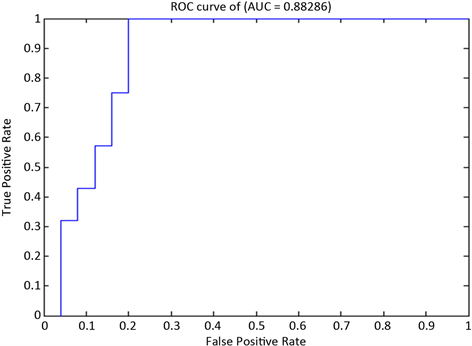﻿ 基于NaiveBayes模型的贵州制造业绩效评价的分类研究

# 基于NaiveBayes模型的贵州制造业绩效评价的分类研究Research on the Classification of Manufacturing Performance Evaluation in Guizhou Based on NaiveBayes Model

Abstract: In this paper, NaiveBayes model is introduced into the classification of performance evaluation of manufacturing industry in Guizhou Province. 529 audited and unqualified financial statement data of manufacturing industry in Guizhou Province from 2014 to 2017 are collected. Combined with the standard value of enterprise performance evaluation published by the state, the performance factors of profitability, operation ability and solvency are quantified by sections. By constructing a series of variables, this paper establishes the financial ability classification model of enterprise performance evaluation, and explores the relationship between the performance evaluation content of manufacturing industry and other subjects of financial statements. Under the evaluation criteria of accuracy and AUC, the results of model training and empirical analysis show that the performance of NaiveBayes model is better than that of logistic regression model, BP neural network and binary SVM and decision tree.

1. 引言

2. NB模型

$P\left({C}_{i}|X\right)\succ P\left({C}_{j}|X\right)，\text{\hspace{0.17em}}1\le j,i\le m,j\ne i$

$P\left({C}_{i}|X\right)=\frac{P\left(X|{C}_{i}\right)P\left({C}_{i}\right)}{P\left(X\right)}$

$P\left(X|{C}_{i}\right)=\underset{k=1}{\overset{n}{\prod }}P\left({X}_{k}|{C}_{i}\right)=P\left({X}_{1}|{C}_{i}\right)P\left({X}_{2}|{C}_{i}\right)\cdots P\left({X}_{n}|{C}_{i}\right)$ (1)

1) 式中 $P\left({X}_{i}|{C}_{i}\right)$ 可由训练样本估计，为了预测X的属类标号，对于每个类 ${C}_{i}$，可以计算出 $P\left(X|{C}_{i}\right)P\left({C}_{i}\right)$。若先验概率 $P\left({C}_{i}\right)$ 未知，可假设 $P\left({C}_{i}\right)$ 相同，此时最大化 $P\left(X|{C}_{i}\right)$ 即可。否则最大化 $P\left(X|{C}_{i}\right)P\left({C}_{i}\right)$$P\left({C}_{i}\right)=\frac{|{C}_{i,D}|}{|D|}$，其中D是训练样本总数， $|{C}_{i,D}|$ 是类 ${C}_{i}$ 在D中的训练元组数。

NB分类法预测输入X的类 ${C}_{i}$，当且仅当

$P\left(X|{C}_{i}\right)P\left({C}_{i}\right)>P\left(X|{C}_{j}\right)P\left({C}_{j}\right)，\text{\hspace{0.17em}}1\le i,j\le m,j\ne i$

$P\left({C}_{i}|X\right)=\mathrm{max}\left\{\underset{k=1}{\overset{n}{\prod }}P\left({X}_{k}|{C}_{i}\right)P\left({C}_{i}\right)\right\}$

3. 模型评价指标

3.1. 准确率(Accuracy)指标

$acc=\mathrm{max}\left\{acc1,acc2,\cdots ,accN\right\}$

3.2. AUC指标Figure 1. ROC curve

4. 变量选取

4.1. 因变量的选取

Table 2. Description of dependent variables

$Y=\left\{\begin{array}{ll}0\hfill & X\le a\hfill \\ 1\hfill & X>a\hfill \end{array}$Table 3. Profitability indicators

$y=\left\{\begin{array}{l}0\text{\hspace{0.17em}}\text{\hspace{0.17em}}\text{\hspace{0.17em}}\text{if}\text{\hspace{0.17em}}0\le x<3\\ 0\text{\hspace{0.17em}}\text{\hspace{0.17em}}\text{\hspace{0.17em}}\text{if}\text{\hspace{0.17em}}3\le x\le 5\end{array}$Table 4. Classification accuracy and effect

4.2. 解释变量选取及说明Table 5. Explanation and meaning in autovariation

5. 实证分析

5.1. 数据来源

5.2. 数据预处理

$x=\frac{{x}_{i}-\stackrel{¯}{x}}{s}$

5.3. 建模结果分析Table 6. Classification results of NB model on profitability indicator test set dataFigure 2. ROC curve of NB classification model on the profitability indicator test setTable 7. Classification results of NB model on operational capacity indicator test set dataFigure 3. ROC curve of NB classification model on operational capacity indicator test setTable 8. Classification results of NB model on solvency indicator test set dataFigure 4. ROC curve of NB classification model on solvency indicator test setTable 9. Empirical results of each performance evaluation classification model

6. 结束语

 王全在. 基于因子分析模型的汽车制造行业绩效评价研究[J]. 会计之友, 2017(23): 25-30.

 陈姿颖. BP神经网络在港口绩效中的应用研究[D]: [硕士学位论文]. 北京: 交通大学, 2015.

 褚淑珍, 杨佳欢. BP神经网络在医药企业绩效评价中的应用——以我国中成药上市企业为例[J]. 新药述评与论坛, 2015, 24(17): 1925-1929.

 朱和平, 郭佳佳. 基于TOPSIS方法的财务绩效发展评价研究——以无锡制造业上市公司为样本[J]. 会计之友, 2017(12): 57-63.

 何清, 陈晓芳. 基于社会经济综合效益的制造业绩效评价研究[J]. 财会通讯, 2012(16): 19-21.

 曾雄旺, 朱敏迪, 杨亦民. 上市农业产业化龙头企业财务绩效评价[J]. 会计之友, 2017(10): 73-77.

 杨小兰, 孙兴. 贵州省制造业信息化年度绩效评价指标体系研究与设计[J]. 科技情报开发与经济, 2009(5): 63-65.

 米妍, 谢瑞峰, 李洁. 资本结构与企业绩效相关性研究——基于中小板制造业上市公司实证分析[J]. 商业会计, 2017(22): 50-53.

 蒙肖莲, 杜宽旗, 杨毓. 企业财务危机预测的贝叶斯模型研究[J]. 数理统计与管理, 2011(6): 1039-1050.

 王润. 东阿阿胶股份有限公司财务报表分析[J]. 河北企业, 2018(8): 88-89.

 国务院国资委财务监督与考核评价局. 企业绩效评价标准值[M]. 北京: 经济科学出版社, 2016: 1-5.

 胡心瀚, 叶五一, 缪柏其. 上市公司信用风险分析模型中的变量选择[J]. 数理统计与管理, 2012, 31(6): 1117-1124.

 Li, R., Zhong, W. and Zhu, L. (2012) Feature Screening via Distance Correlation Learning. Journal of the American Statistical Association, 107, 1129-1139.

Top